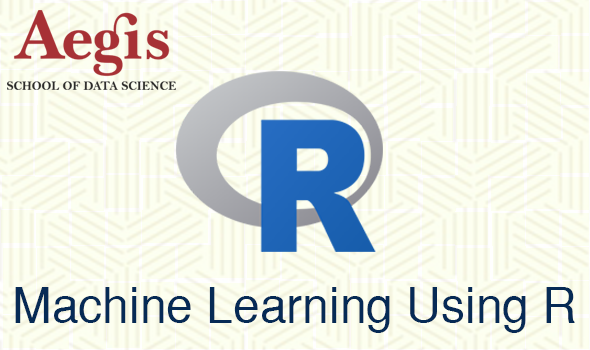#### Machine Learning using RApplication fee : 13.7 USD

#### Details

Location: On-campus,Online Certificate course Mr. Ritin Joshi English 205.53 USD 18% 242.53 USD ★★★★★No Ratings

# Course Details

“Data is a precious thing and will last longer than the systems themselves.”

– Tim Berners-Lee, inventor of the World Wide Web.

THE OBJECTIVE OF THIS COURSE IS TO GIVE STUDENTS:

• basic knowledge about the key algorithms and theory that form the foundation of machine learning and computational intelligence
• A practical knowledge of machine learning algorithms and methods so that they will be able to explain the principles, advantages, limitations such as over fitting and possible applications of machine learning
• Identify and apply the appropriate machine learning technique to Regression, Classification, Clustering problems.

BACKGROUND:

Do you have data and wonder what it can tell you?  Do you need a deeper understanding of the core ways in which machine learning can improve your business?  Do you want to be able to converse with specialists about anything from regression and classification to deep learning and recommender systems?

Machine learning is the heart of Data Science. Data science is mainly focused on the use of machine learning for solving problems. This course will cover the basic components of building and applying prediction functions with an emphasis on practical applications. The course will provide basic grounding in concepts such as training and tests sets, overfitting, and error rates. The course will also introduce a range of model based and algorithmic machine learning methods including Regression, Classification, and Clustering.

Why with R???

R is one of the most highly regarded, highly ranked, and fastest growing data language. To understand why this is true, let’s look at the results of several important surveys and programming language rankings to see where R shakes out:

IEEE: R ranks #5

TIOBE: R ranks high with consistent upward trend

Redmonk: R is #12

O’Reilly: R is arguably the most common data programming language

LEARNING OUTCOME:

In this course, you will get hands-on experience with machine learning from a series of practical case-studies. Through hands-on practice, you will be able to apply machine learning methods in a wide range of domains. Machine learning is the key skill for the jobs of Data scientist, Data Analysis. After completion of the course, candidate will be eligible for the above mention jobs.

TEACHING METHODOLOGY:

Teaching Methodology consists of theory and practical with interactive discussions on every topic. Presentation and board teaching on smart board make it easy to understand for the candidate. After class, assessment would be in the form MCQs, assignments etc.

PRE-REQUISITES:

Fundamentals of programming, Statistics and Probability, Basic knowledge of R language.

COURSE OUTLINE:

1. Introduction
• Data Science, Big data
• Predictive Analysis, Machine Learning, Data Mining, Soft computing
• Neural Network, Deep learning
• Introduction to Machine learning: Machine Learning Basics, how machines learn, Machine learning in practice, Types of Machine Learning.
2. Data Preparation
• Data Collection
• Outlier Detection and Treatment
• Missing Value/NA Imputation Techniques
• Dividing Data into Training, Testing and Validation Sets
3. Regression: Model Building and Validation of Model
• Which kind of the problems can be solved using this family of algorithms
• How to interpret the output.
• Simple Linear Regression(SLR), Model building with SLR, Validation of Model
• Multiple Linear Regression(MLR), Model building with MLR, Validation of Model
• Gradient Descent Algorithm, Linear Discriminant Analysis, Factor Analysis
4. Classification: Model Building and Validation of Model
• Which kind of the problems can be solved using this family of algorithms
• How to interpret the output/ validation matrix
• Logistic Regression(LR) for Classification, Model building with LR, Validation of Model
• Decision Trees and Random Forest, Model building with it, Validation of Model
• K-Nearest Neighbours Classifier, Model building with it, Validation of Model
• Naïve Bayes Classifier, Model building with it, Validation of Model
• Support Vector Machine Classifier, Model building with it, Validation of Model
• How to interpret output or validation matrix
5. Clustering: Model Building and Validation of Model
• Which kind of the problems can be solved using this family of algorithms
• How to interpret the output/ validation matrix
• Clustering as Machine Learning Task
• K-means Clustering Algorithm
• Model building with K- Means and Validation of Models.
6. Model Selection and Deployment
• Cross validation
• Comparing Results of different Algorithm and selecting the best Algorithm.
7. Time Series Analysis
8. Recommendation System

TOOLS/SOFTWARE:

R             https://cran.r-project.org/bin/windows/base/